Courses

# Olympiad Test : Embedded Figures

## 20 Questions MCQ Test Science Olympiad for Class 5 | Olympiad Test : Embedded Figures

Description
This mock test of Olympiad Test : Embedded Figures for Class 5 helps you for every Class 5 entrance exam. This contains 20 Multiple Choice Questions for Class 5 Olympiad Test : Embedded Figures (mcq) to study with solutions a complete question bank. The solved questions answers in this Olympiad Test : Embedded Figures quiz give you a good mix of easy questions and tough questions. Class 5 students definitely take this Olympiad Test : Embedded Figures exercise for a better result in the exam. You can find other Olympiad Test : Embedded Figures extra questions, long questions & short questions for Class 5 on EduRev as well by searching above.
QUESTION: 1

Solution:
QUESTION: 2

Solution:
QUESTION: 3

### One fig (X) is given that is embedded in any one of the four alternatives figures (A), (B), (C) and (D). Find the alternative which contains figure (X) as its part.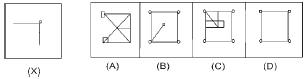Solution:
QUESTION: 4

One fig (X) is given that is embedded in any one of the four alternatives figures (A), (B), (C) and (D). Find the alternative which contains figure (X) as its part.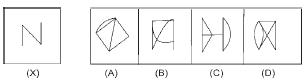Solution:
QUESTION: 5

One fig (X) is given that is embedded in any one of the four alternatives figures (A), (B), (C) and (D). Find the alternative which contains figure (X) as its part.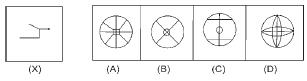Solution:
QUESTION: 6

One fig (X) is given that is embedded in any one of the four alternatives figures (A), (B), (C) and (D). Find the alternative which contains figure (X) as its part.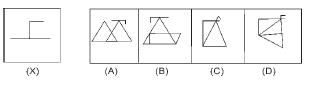Solution:
QUESTION: 7

One fig (X) is given that is embedded in any one of the four alternatives figures (A), (B), (C) and (D). Find the alternative which contains figure (X) as its part.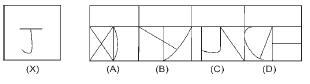Solution:
QUESTION: 8

One fig (X) is given that is embedded in any one of the four alternatives figures (A), (B), (C) and (D). Find the alternative which contains figure (X) as its part.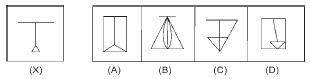Solution:
QUESTION: 9

One fig (X) is given that is embedded in any one of the four alternatives figures (A), (B), (C) and (D). Find the alternative which contains figure (X) as its part.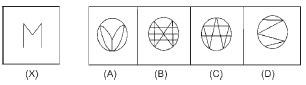Solution:
QUESTION: 10

One fig (X) is given that is embedded in any one of the four alternatives figures (A), (B), (C) and (D). Find the alternative which contains figure (X) as its part.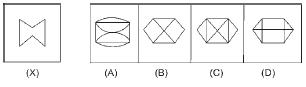Solution:
QUESTION: 11

One fig (X) is given that is embedded in any one of the four alternatives figures (A), (B), (C) and (D). Find the alternative which contains figure (X) as its part.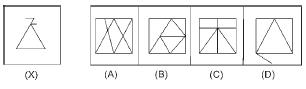Solution:
QUESTION: 12

One fig (X) is given that is embedded in any one of the four alternatives figures (A), (B), (C) and (D). Find the alternative which contains figure (X) as its part.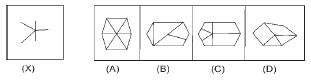Solution:
QUESTION: 13

One fig (X) is given that is embedded in any one of the four alternatives figures (A), (B), (C) and (D). Find the alternative which contains figure (X) as its part.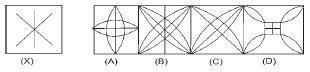Solution:
QUESTION: 14

One fig (X) is given that is embedded in any one of the four alternatives figures (A), (B), (C) and (D). Find the alternative which contains figure (X) as its part.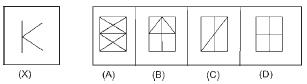Solution:
QUESTION: 15

In the following questions, choose the alternative figure which is embedded in the given Figure (X).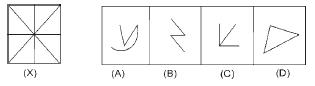Solution:
QUESTION: 16

In the following questions, choose the alternative figure which is embedded in the given Figure (X).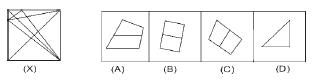Solution:
QUESTION: 17

In the following questions, choose the alternative figure which is embedded in the given Figure (X).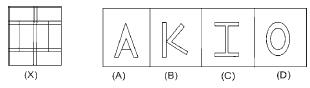Solution:
QUESTION: 18

In the following questions, choose the alternative figure which is embedded in the given Figure (X).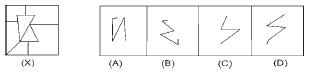Solution:
QUESTION: 19

In the following questions, choose the alternative figure which is embedded in the given Figure (X).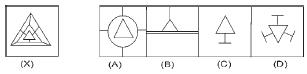Solution:
QUESTION: 20

In the following questions, choose the alternative figure which is embedded in the given Figure (X).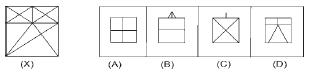Solution: